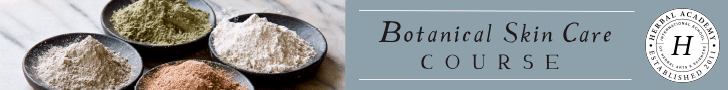# Equivalents

 Dosage Equivalents 1 teaspoon = 5 ml 1/2 teaspoon = 2.5 ml 1/4 teaspoon = 1.25 ml 1/5 teaspoon = 1 ml 1/8 teaspoon = .616 ml 100 drops = 1 teaspoon 50 drops = 1/2 teaspoon 25 drops = 1/4 teaspoon 12 drops = 1/8 teaspoon 1 fl oz = approx 30 ml 2 fl oz = approx 59 ml 3 fl oz = approx 89 ml 4 fl oz = approx 118 ml 8 fl oz = approx 237 ml 1 fl oz = 6 teaspoons 2 fl oz = 12 teaspoons 3 fl oz = 18 teaspoons 4 fl oz = 24 teaspoons 5 fl oz = 30 teaspoons 6 fl oz = 36 teaspoons 7 fl oz = 42 teaspoons 8 fl oz = 48 teaspoons 1 ml = 20 drops 2 ml = 40 drops 3 ml = 60 drops conversions from U.S. to metric may be approximate

 Weights & Measurement Equivalents Length 12 inches = 1 foot 3 feet = 1 yard 220 yards = 1 furlong 8 furlongs = 1 mile 5280 feet = 1 mile 1760 yards = 1 mile Area 144 sq. inches = 1 square foot 9 sq. feet = 1 square yard 43,560 square feet = 1 acre 4840 square yards = 1 acre 640 acres = 1 square mile 1 sq.mile = 1 section 36 sections = 1 township Volume 1728 cu. inches = 1 cubic foot 27 cu. feet = 1 cubic yard Capacity (Liquid) 16 fluid ounces = 1 pint 4 gills = 1 pint 2 pints = 1 quart 4 quarts = 1 gallon 8 pints = 1 gallon Capacity (dry) 16 fluid ounces = 1 pint 2 pints = 1 quart 8 quarts = 1 peck 4 pecks = 1 bushel Mass/Weight 437.5 grains = 1 ounce 16 ounces = 1 pound (7000 grains) 14 pounds = 1 stone 100 pounds = 1 hundredweight [cwt] 20 cwt = 1 ton (2000 pounds) Troy Weights 24 grains = 1 pennyweight 20 pennyweights = 1 ounce (480 grains) 12 ounces = 1 pound (5760 grains) Apothecaries’ Measures 60 minims = 1 fl.dram 8 fl.drams = 1 fl. ounce 16 fl. ounces = 1 pint Apothecaries’ Weights 20 grains = 1 scruple 3 scruples = 1 dram 8 drams = 1 ounce (480 grains) 12 ounces = 1 pound (5760 grains)

 Cooking Measurement Equivalents (US) 1 teaspoon = 1/6 fl. ounce 1 Tablespoon = 1/2 fl. ounce 1 Tablespoon = 3 teaspoons 1/16 cup = 1 Tablespoon 1/8 cup = 2 Tablespoons 1/6 cup = 2 Tablespoons + 2 teaspoons 1/4 cup = 4 Tablespoons 1/3 cup = 5 Tablespoons + 1 teaspoon 3/8 cup = 6 Tablespoons 1/2 cup = 8 Tablespoons 2/3 cup = 10 Tablespoons + 2 teaspoons 3/4 cup = 12 Tablespoons 1 cup = 48 teaspoons 1 cup = 16 Tablespoons 1 cup = 8 fluid ounces 2 cups = 1 pint 2 cups = 16 fluid ounces 4 cups = 1 quart 4 cups = 32 fluid ounces 8 cups = 4 pints 8 cups = 1/2 gallon 8 cups = 64 fluid ounces 1 quart = 2 pints 4 quarts = 1 gallon 4 quarts = 128 fluid ounces 1 gallon = 4 quarts 16 ounces = 1 pound